## 7.2 Virtual sampling

In the previous Section 7.1, we performed a tactile sampling activity by hand. In other words, we used a physical bowl of balls and a physical shovel. We performed this sampling activity by hand first so that we could develop a firm understanding of the root ideas behind sampling. In this section, we’ll mimic this tactile sampling activity with a virtual sampling activity using a computer. In other words, we’ll use a virtual analog to the bowl of balls and a virtual analog to the shovel.

### 7.2.1 Using the virtual shovel once

Let’s start by performing the virtual analog of the tactile sampling exercise we performed in Section 7.1. We first need a virtual analog of the bowl seen in Figure 7.1. To this end, we included a data frame named bowl in the moderndive package. The rows of bowl correspond exactly with the contents of the actual bowl.

bowl
# A tibble: 2,400 x 2
ball_ID color
<int> <chr>
1       1 white
2       2 white
3       3 white
4       4 red
5       5 white
6       6 white
7       7 red
8       8 white
9       9 red
10      10 white
# … with 2,390 more rows

Observe that bowl has 2400 rows, telling us that the bowl contains 2400 equally sized balls. The first variable ball_ID is used as an identification variable as discussed in Subsection 1.4.4; none of the balls in the actual bowl are marked with numbers. The second variable color indicates whether a particular virtual ball is red or white. View the contents of the bowl in RStudio’s data viewer and scroll through the contents to convince yourself that bowl is indeed a virtual analog of the actual bowl in Figure 7.1.

Now that we have a virtual analog of our bowl, we now need a virtual analog to the shovel seen in Figure 7.2 to generate virtual samples of 50 balls. We’re going to use the rep_sample_n() function included in the moderndive package. This function allows us to take repeated, or replicated, samples of size n.

virtual_shovel <- bowl %>%
rep_sample_n(size = 50)
virtual_shovel
# A tibble: 50 x 3
# Groups:   replicate 
replicate ball_ID color
<int>   <int> <chr>
1         1    1970 white
2         1     842 red
3         1    2287 white
4         1     599 white
5         1     108 white
6         1     846 red
7         1     390 red
8         1     344 white
9         1     910 white
10         1    1485 white
# … with 40 more rows

Observe that virtual_shovel has 50 rows corresponding to our virtual sample of size 50. The ball_ID variable identifies which of the 2400 balls from bowl are included in our sample of 50 balls while color denotes its color. However, what does the replicate variable indicate? In virtual_shovel’s case, replicate is equal to 1 for all 50 rows. This is telling us that these 50 rows correspond to the first repeated/replicated use of the shovel, in our case our first sample. We’ll see shortly that when we “virtually” take 33 samples, replicate will take values between 1 and 33.

Let’s compute the proportion of balls in our virtual sample that are red using the dplyr data wrangling verbs you learned in Chapter 3. First, for each of our 50 sampled balls, let’s identify if it is red or not using a test for equality with ==. Let’s create a new Boolean variable is_red using the mutate() function from Section 3.5:

virtual_shovel %>%
mutate(is_red = (color == "red"))
# A tibble: 50 x 4
# Groups:   replicate 
replicate ball_ID color is_red
<int>   <int> <chr> <lgl>
1         1    1970 white FALSE
2         1     842 red   TRUE
3         1    2287 white FALSE
4         1     599 white FALSE
5         1     108 white FALSE
6         1     846 red   TRUE
7         1     390 red   TRUE
8         1     344 white FALSE
9         1     910 white FALSE
10         1    1485 white FALSE
# … with 40 more rows

Observe that for every row where color == "red", the Boolean (logical) value TRUE is returned and for every row where color is not equal to "red", the Boolean FALSE is returned.

Second, let’s compute the number of balls out of 50 that are red using the summarize() function. Recall from Section 3.3 that summarize() takes a data frame with many rows and returns a data frame with a single row containing summary statistics, like the mean() or median(). In this case, we use the sum():

virtual_shovel %>%
mutate(is_red = (color == "red")) %>%
summarize(num_red = sum(is_red))
# A tibble: 1 x 2
replicate num_red
<int>   <int>
1         1      12

Why does this work? Because R treats TRUE like the number 1 and FALSE like the number 0. So summing the number of TRUEs and FALSEs is equivalent to summing 1’s and 0’s. In the end, this operation counts the number of balls where color is red. In our case, 12 of the 50 balls were red. However, you might have gotten a different number red because of the randomness of the virtual sampling.

Third and lastly, let’s compute the proportion of the 50 sampled balls that are red by dividing num_red by 50:

virtual_shovel %>%
mutate(is_red = color == "red") %>%
summarize(num_red = sum(is_red)) %>%
mutate(prop_red = num_red / 50)
# A tibble: 1 x 3
replicate num_red prop_red
<int>   <int>    <dbl>
1         1      12     0.24

In other words, 24% of this virtual sample’s balls were red. Let’s make this code a little more compact and succinct by combining the first mutate() and the summarize() as follows:

virtual_shovel %>%
summarize(num_red = sum(color == "red")) %>%
mutate(prop_red = num_red / 50)
# A tibble: 1 x 3
replicate num_red prop_red
<int>   <int>    <dbl>
1         1      12     0.24

Great! 24% of virtual_shovel’s 50 balls were red! So based on this particular sample of 50 balls, our guess at the proportion of the bowl’s balls that are red is 24%. But remember from our earlier tactile sampling activity that if we repeat this sampling, we will not necessarily obtain the same value of 24% again. There will likely be some variation. In fact, our 33 groups of friends computed 33 such proportions whose distribution we visualized in Figure 7.6. We saw that these estimates varied. Let’s now perform the virtual analog of having 33 groups of students use the sampling shovel!

### 7.2.2 Using the virtual shovel 33 times

Recall that in our tactile sampling exercise in Section 7.1, we had 33 groups of students each use the shovel, yielding 33 samples of size 50 balls. We then used these 33 samples to compute 33 proportions. In other words, we repeated/replicated using the shovel 33 times. We can perform this repeated/replicated sampling virtually by once again using our virtual shovel function rep_sample_n(), but by adding the reps = 33 argument. This is telling R that we want to repeat the sampling 33 times.

We’ll save these results in a data frame called virtual_samples. While we provide a preview of the first 10 rows of virtual_samples in what follows, we highly suggest you scroll through its contents using RStudio’s spreadsheet viewer by running View(virtual_samples).

virtual_samples <- bowl %>%
rep_sample_n(size = 50, reps = 33)
virtual_samples
# A tibble: 1,650 x 3
# Groups:   replicate 
replicate ball_ID color
<int>   <int> <chr>
1         1     875 white
2         1    1851 red
3         1    1548 red
4         1    1975 white
5         1     835 white
6         1      16 white
7         1     327 white
8         1    1803 red
9         1     740 red
10         1     179 red
# … with 1,640 more rows

Observe in the spreadsheet viewer that the first 50 rows of replicate are equal to 1 while the next 50 rows of replicate are equal to 2. This is telling us that the first 50 rows correspond to the first sample of 50 balls while the next 50 rows correspond to the second sample of 50 balls. This pattern continues for all reps = 33 replicates and thus virtual_samples has 33 $$\cdot$$ 50 = 1650 rows.

Let’s now take virtual_samples and compute the resulting 33 proportions red. We’ll use the same dplyr verbs as before, but this time with an additional group_by() of the replicate variable. Recall from Section 3.4 that by assigning the grouping variable “meta-data” before we summarize(), we’ll obtain 33 different proportions red. We display a preview of the first 10 out of 33 rows:

virtual_prop_red <- virtual_samples %>%
group_by(replicate) %>%
summarize(red = sum(color == "red")) %>%
mutate(prop_red = red / 50)
virtual_prop_red
# A tibble: 33 x 3
replicate   red prop_red
<int> <int>    <dbl>
1         1    23     0.46
2         2    19     0.38
3         3    18     0.36
4         4    19     0.38
5         5    15     0.3
6         6    21     0.42
7         7    21     0.42
8         8    16     0.32
9         9    24     0.48
10        10    14     0.28
# … with 23 more rows

As with our 33 groups of friends’ tactile samples, there is variation in the resulting 33 virtual proportions red. Let’s visualize this variation in a histogram in Figure 7.8. Note that we add binwidth = 0.05 and boundary = 0.4 arguments as well. Recall that setting boundary = 0.4 ensures a binning scheme with one of the bins’ boundaries at 0.4. Since the binwidth = 0.05 is also set, this will create bins with boundaries at 0.30, 0.35, 0.45, 0.5, etc. as well.

ggplot(virtual_prop_red, aes(x = prop_red)) +
geom_histogram(binwidth = 0.05, boundary = 0.4, color = "white") +
labs(x = "Proportion of 50 balls that were red",
title = "Distribution of 33 proportions red")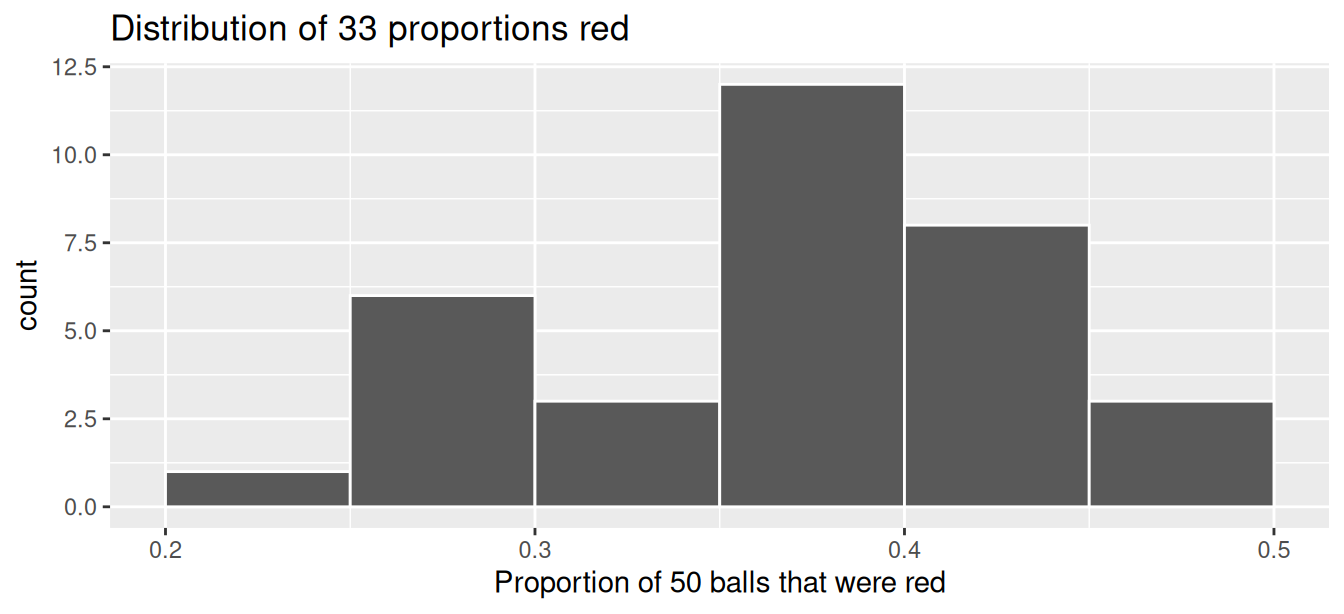FIGURE 7.8: Distribution of 33 proportions based on 33 samples of size 50.

Observe that we occasionally obtained proportions red that are less than 30%. On the other hand, we occasionally obtained proportions that are greater than 45%. However, the most frequently occurring proportions were between 35% and 40% (for 11 out of 33 samples). Why do we have these differences in proportions red? Because of sampling variation.

Let’s now compare our virtual results with our tactile results from the previous section in Figure 7.9. Observe that both histograms are somewhat similar in their center and variation, although not identical. These slight differences are again due to random sampling variation. Furthermore, observe that both distributions are somewhat bell-shaped.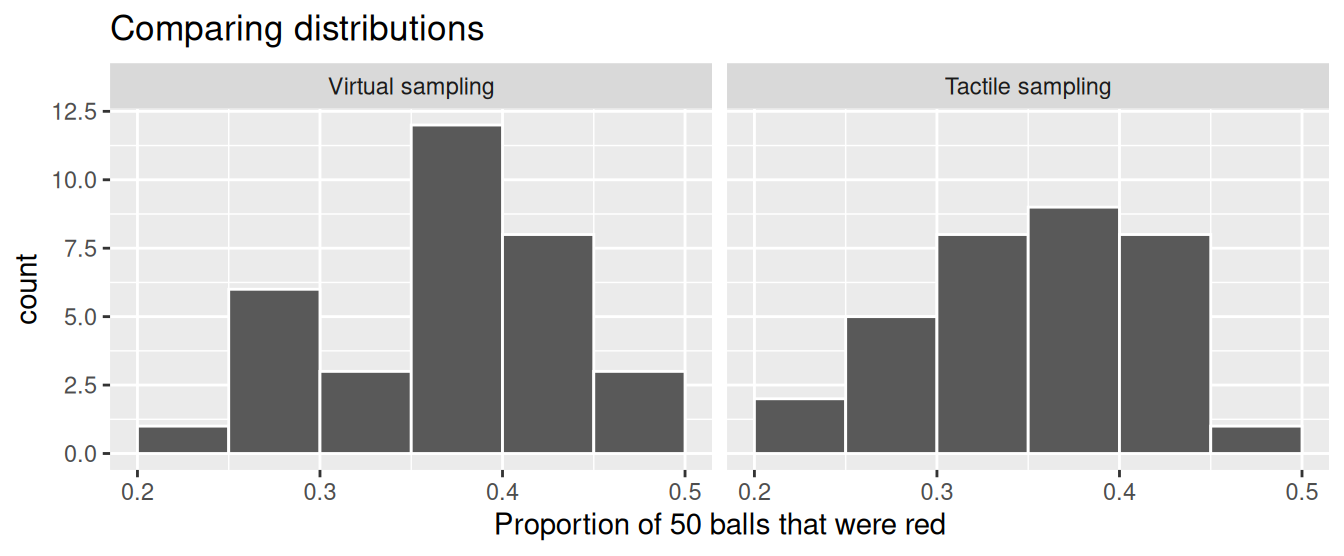FIGURE 7.9: Comparing 33 virtual and 33 tactile proportions red.

Learning check

(LC7.3) Why couldn’t we study the effects of sampling variation when we used the virtual shovel only once? Why did we need to take more than one virtual sample (in our case 33 virtual samples)?

### 7.2.3 Using the virtual shovel 1000 times

Now say we want to study the effects of sampling variation not for 33 samples, but rather for a larger number of samples, say 1000. We have two choices at this point. We could have our groups of friends manually take 1000 samples of 50 balls and compute the corresponding 1000 proportions. However, this would be a tedious and time-consuming task. This is where computers excel: automating long and repetitive tasks while performing them quite quickly. Thus, at this point we will abandon tactile sampling in favor of only virtual sampling. Let’s once again use the rep_sample_n() function with sample size set to be 50 once again, but this time with the number of replicates reps set to 1000. Be sure to scroll through the contents of virtual_samples in RStudio’s viewer.

virtual_samples <- bowl %>%
rep_sample_n(size = 50, reps = 1000)
virtual_samples
# A tibble: 50,000 x 3
# Groups:   replicate [1,000]
replicate ball_ID color
<int>   <int> <chr>
1         1    1236 red
2         1    1944 red
3         1    1939 white
4         1     780 white
5         1    1956 white
6         1    1003 white
7         1    2113 white
8         1    2213 white
9         1     782 white
10         1     898 white
# … with 49,990 more rows

Observe that now virtual_samples has 1000 $$\cdot$$ 50 = 50,000 rows, instead of the 33 $$\cdot$$ 50 = 1650 rows from earlier. Using the same data wrangling code as earlier, let’s take the data frame virtual_samples with 1000 $$\cdot$$ 50 = 50,000 rows and compute the resulting 1000 proportions of red balls.

virtual_prop_red <- virtual_samples %>%
group_by(replicate) %>%
summarize(red = sum(color == "red")) %>%
mutate(prop_red = red / 50)
virtual_prop_red
# A tibble: 1,000 x 3
replicate   red prop_red
<int> <int>    <dbl>
1         1    18     0.36
2         2    19     0.38
3         3    20     0.4
4         4    15     0.3
5         5    17     0.34
6         6    16     0.32
7         7    23     0.46
8         8    23     0.46
9         9    15     0.3
10        10    18     0.36
# … with 990 more rows

Observe that we now have 1000 replicates of prop_red, the proportion of 50 balls that are red. Using the same code as earlier, let’s now visualize the distribution of these 1000 replicates of prop_red in a histogram in Figure 7.10.

ggplot(virtual_prop_red, aes(x = prop_red)) +
geom_histogram(binwidth = 0.05, boundary = 0.4, color = "white") +
labs(x = "Proportion of 50 balls that were red",
title = "Distribution of 1000 proportions red")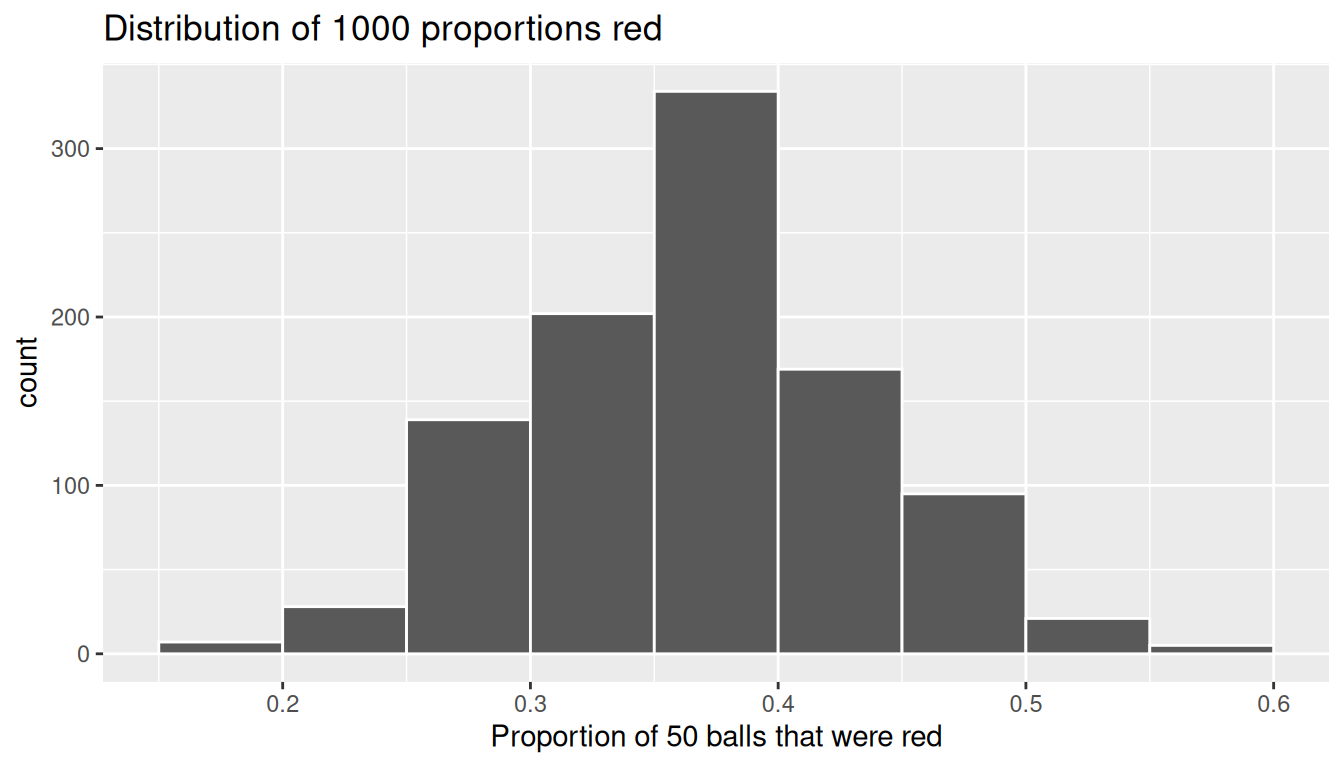FIGURE 7.10: Distribution of 1000 proportions based on 1000 samples of size 50.

Once again, the most frequently occurring proportions of red balls occur between 35% and 40%. Every now and then, we obtain proportions as low as between 20% and 25%, and others as high as between 55% and 60%. These are rare, however. Furthermore, observe that we now have a much more symmetric and smoother bell-shaped distribution. This distribution is, in fact, approximated well by a normal distribution. At this point we recommend you read the “Normal distribution” section (Appendix A.2) for a brief discussion on the properties of the normal distribution.

Learning check

(LC7.4) Why did we not take 1000 “tactile” samples of 50 balls by hand?

(LC7.5) Looking at Figure 7.10, would you say that sampling 50 balls where 30% of them were red is likely or not? What about sampling 50 balls where 10% of them were red?

### 7.2.4 Using different shovels

Now say instead of just one shovel, you have three choices of shovels to extract a sample of balls with: shovels of size 25, 50, and 100.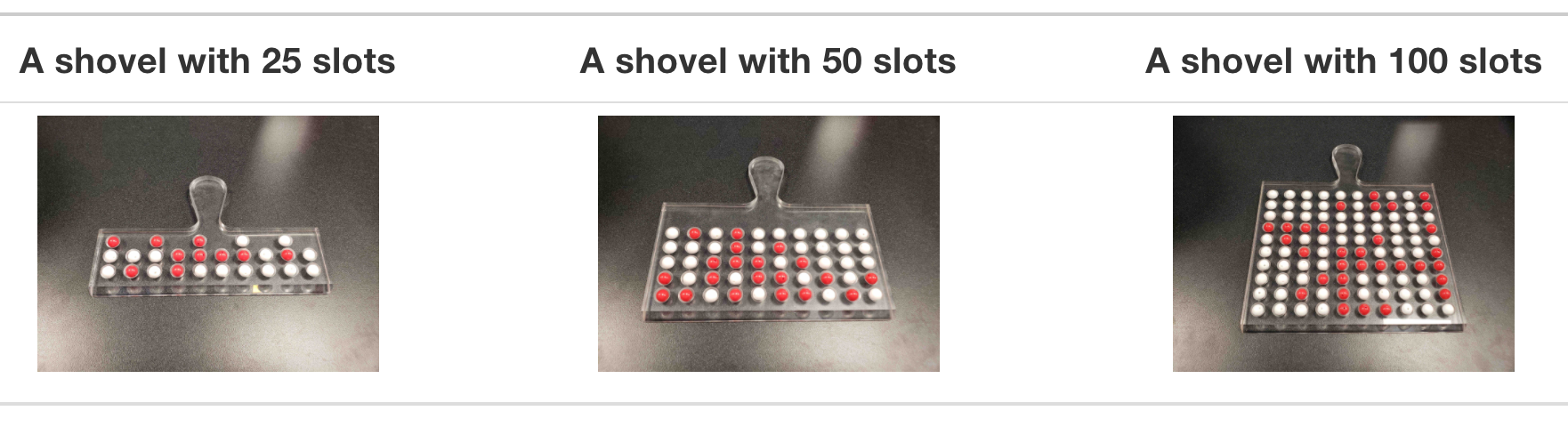FIGURE 7.11: Three shovels to extract three different sample sizes.

If your goal is still to estimate the proportion of the bowl’s balls that are red, which shovel would you choose? In our experience, most people would choose the largest shovel with 100 slots because it would yield the “best” guess of the proportion of the bowl’s balls that are red. Let’s define some criteria for “best” in this subsection.

Using our newly developed tools for virtual sampling, let’s unpack the effect of having different sample sizes! In other words, let’s use rep_sample_n() with size set to 25, 50, and 100, respectively, while keeping the number of repeated/replicated samples at 1000:

1. Virtually use the appropriate shovel to generate 1000 samples with size balls.
2. Compute the resulting 1000 replicates of the proportion of the shovel’s balls that are red.
3. Visualize the distribution of these 1000 proportions red using a histogram.

Run each of the following code segments individually and then compare the three resulting histograms.

# Segment 1: sample size = 25 ------------------------------
# 1.a) Virtually use shovel 1000 times
virtual_samples_25 <- bowl %>%
rep_sample_n(size = 25, reps = 1000)

# 1.b) Compute resulting 1000 replicates of proportion red
virtual_prop_red_25 <- virtual_samples_25 %>%
group_by(replicate) %>%
summarize(red = sum(color == "red")) %>%
mutate(prop_red = red / 25)

# 1.c) Plot distribution via a histogram
ggplot(virtual_prop_red_25, aes(x = prop_red)) +
geom_histogram(binwidth = 0.05, boundary = 0.4, color = "white") +
labs(x = "Proportion of 25 balls that were red", title = "25")

# Segment 2: sample size = 50 ------------------------------
# 2.a) Virtually use shovel 1000 times
virtual_samples_50 <- bowl %>%
rep_sample_n(size = 50, reps = 1000)

# 2.b) Compute resulting 1000 replicates of proportion red
virtual_prop_red_50 <- virtual_samples_50 %>%
group_by(replicate) %>%
summarize(red = sum(color == "red")) %>%
mutate(prop_red = red / 50)

# 2.c) Plot distribution via a histogram
ggplot(virtual_prop_red_50, aes(x = prop_red)) +
geom_histogram(binwidth = 0.05, boundary = 0.4, color = "white") +
labs(x = "Proportion of 50 balls that were red", title = "50")

# Segment 3: sample size = 100 ------------------------------
# 3.a) Virtually using shovel with 100 slots 1000 times
virtual_samples_100 <- bowl %>%
rep_sample_n(size = 100, reps = 1000)

# 3.b) Compute resulting 1000 replicates of proportion red
virtual_prop_red_100 <- virtual_samples_100 %>%
group_by(replicate) %>%
summarize(red = sum(color == "red")) %>%
mutate(prop_red = red / 100)

# 3.c) Plot distribution via a histogram
ggplot(virtual_prop_red_100, aes(x = prop_red)) +
geom_histogram(binwidth = 0.05, boundary = 0.4, color = "white") +
labs(x = "Proportion of 100 balls that were red", title = "100") 

For easy comparison, we present the three resulting histograms in a single row with matching x and y axes in Figure 7.12.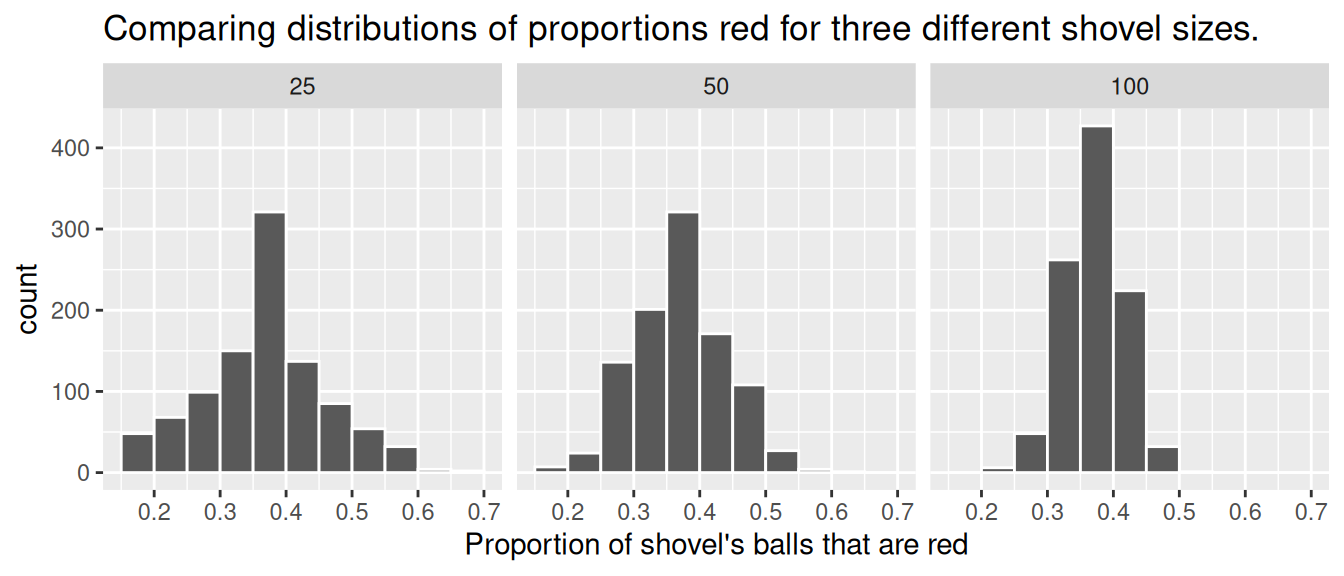FIGURE 7.12: Comparing the distributions of proportion red for different sample sizes.

Observe that as the sample size increases, the variation of the 1000 replicates of the proportion of red decreases. In other words, as the sample size increases, there are fewer differences due to sampling variation and the distribution centers more tightly around the same value. Eyeballing Figure 7.12, all three histograms appear to center around roughly 40%.

We can be numerically explicit about the amount of variation in our three sets of 1000 values of prop_red using the standard deviation. A standard deviation is a summary statistic that measures the amount of variation within a numerical variable (see Appendix A.1 for a brief discussion on the properties of the standard deviation). For all three sample sizes, let’s compute the standard deviation of the 1000 proportions red by running the following data wrangling code that uses the sd() summary function.

# n = 25
virtual_prop_red_25 %>%
summarize(sd = sd(prop_red))

# n = 50
virtual_prop_red_50 %>%
summarize(sd = sd(prop_red))

# n = 100
virtual_prop_red_100 %>%
summarize(sd = sd(prop_red))

Let’s compare these three measures of distributional variation in Table 7.1.

TABLE 7.1: Comparing standard deviations of proportions red for three different shovels
Number of slots in shovel Standard deviation of proportions red
25 0.094
50 0.069
100 0.045

As we observed in Figure 7.12, as the sample size increases, the variation decreases. In other words, there is less variation in the 1000 values of the proportion red. So as the sample size increases, our guesses at the true proportion of the bowl’s balls that are red get more precise.

Learning check

(LC7.6) In Figure 7.12, we used shovels to take 1000 samples each, computed the resulting 1000 proportions of the shovel’s balls that were red, and then visualized the distribution of these 1000 proportions in a histogram. We did this for shovels with 25, 50, and 100 slots in them. As the size of the shovels increased, the histograms got narrower. In other words, as the size of the shovels increased from 25 to 50 to 100, did the 1000 proportions

• A. vary less,
• B. vary by the same amount, or
• C. vary more?

(LC7.7) What summary statistic did we use to quantify how much the 1000 proportions red varied?

• A. The interquartile range
• B. The standard deviation
• C. The range: the largest value minus the smallest.